# Classroom

Of the 26 pupils in the classroom, 12 boys and 14 girls, four representatives are picked to the odds of being:
a) all the girls
b) three girls and one boy
c) there will be at least two boys

p1 =  0.067
p2 =  0.2922
p3 =  0.6409

### Step-by-step explanation:

${p}_{3}=1-{p}_{1}-{p}_{2}=1-0.067-0.2922=0.6409$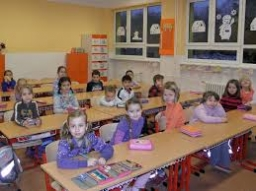Did you find an error or inaccuracy? Feel free to write us. Thank you!Tips to related online calculators
Would you like to compute count of combinations?

## Related math problems and questions:

• Boys and girlsThere are eight boys and nine girls in the class. There were six children on the trip from this class. What is the probability that left a) only boys b) just two boys
• School parliament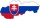There are 18 boys and 14 girls in the class. In how many ways can 3 representatives be elected to the school parliament, if these are to be: a) the boys themselves b) one boy and two girls
• Boys and girlsThere are 11 boys and 18 girls in the classroom. Three pupils will answer. What is the probability that two boys will be among them?There are 15 boys and 12 girls at the graduation party. Determine how many four couples can be selected.
• Peaches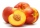There are 20 peaches in the pocket. 3 peaches are rotten. What is the probability that one of the randomly picked two peaches will be just one rotten?
• Created trioWhat is the probability that in the created trio, which consists of 19 boys and 12 girls, they will be: a) the boys themselves b) the girls themselves c) 2 boys and 1 girl?
• In the classroomThere are 30 boys and a few girls in the class. In the six months, 28 boys and all girls benefited, which was 95% of all pupils. How many pupils are there in the classroom?
• Four-member teamThere are 14 girls and 11 boys in the class. How many ways can a four-member team be chosen so that there are exactly two boys in it?
• Families 2Seven hundred twenty-nine families are having six children each. The probability of a girl is 1/3, and the probability of a boy is 2/3. Find the number of families having two girls and four boys.
• Competition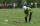15 boys and 10 girls are in the class. On school competition of them is selected 6-member team composed of 4 boys and 2 girls. How many ways can we select students?
• Desks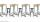A class has 20 students. The classroom consists of 20 desks, with 4 desks in each of 5 different rows. Amy, Bob, Chloe, and David are all friends, and would like to sit in the same row. How many possible seating arrangements are there such that Amy, Bob,
• Boys and girlsThere are 20 boys and 10 girls in the class. How many different dance pairs can we make of them?
• GirlsThe boys and girls in the class formed groups without the rest of the fives, two girls and three boys. There are six girls missing to create mixed pairs (1 boy and one girl). How many girls are in the classroom?
• Family94 boys are born per 100 girls. Determine the probability that there are two boys in a randomly selected family with three children.
• Research in schoolFor particular research in high school, four pupils are to be selected from a class with 30 pupils. Calculate the number of all possible results of the select and further calculate the number of all possible results, if it depends on the order in which th
• Pairs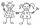From the five girls and four boys teachers have to choose one pair of boy and girl. A) How many such pairs of (M + F)? B) How many pairs where only boys (M + M)? C) How many are all possible pairs?
• Two groupsThe group of 10 girls should be divided into two groups with at least 4 girls in each group. How many ways can this be done?